# Data Transmission and Reception in Spatial Modulation MIMO Wireless Systems and Analysis in Nakagami-m Fading Channels

Data Transmission and Reception in Spatial Modulation MIMO Wireless Systems and Analysis in Nakagami-m Fading Channels

Department of Electronics and Communication Engineering, Panimalar Engineering College, Chennai 600123, Tamil Nadu, India

Department of Electronics Engineering, Dr. Ambedkar Institute of Technology for Handicapped, Kanpur 208024, Uttar Pradesh, India

Corresponding Author Email:
ermpremkumar@yahoo.co.in
Page:
401-406
|
DOI:
https://doi.org/10.18280/ts.400142
2 November 2022
|
Accepted:
9 February 2023
|
Published:
28 February 2023
| Citation

OPEN ACCESS

Abstract:

This research paper provides binary data transmission in spatial modulation (SM) multiple input multiple output (MIMO) wireless systems, its reception in Nakagami-m fading channel and its analysis by pairwise error probability. In spatial modulation MIMO systems data is transmitted through the selected antenna via its index where data bits are sent via Nakagami-m fading channel whenever there is large cluster of multipath waves and large delay period. In the receiver data bits are detected using optimal detection or suboptimal detection from the corresponding antenna index where data bits are separately detected or simultaneously detected. Simulations are performed in matrix laboratory (MATLAB) for different values of modulation order M, number of transmitting antennas Nt, number of receiving antennas Nr and shape parameter m or the fading parameter. Results obtained for simulation of spatial modulation MIMO system shows that the performance in terms of pairwise error probability degrades as the order of modulation increases. However, the fact is that in spatial modulation MIMO system since antenna index is selected for transmission, the number of radio frequency (RF) chain decreases which results in reducing hardware complexity. The obtained results can be useful for research perspective for 5G and 6G wireless systems to cater the requirements of data transmission and reception.

Keywords:

spatial modulation, multiple input multiple output system, Nakagami-m fading, pairwise error probability

1. Introduction

Increase of data traffic in wireless communication, is catered by employing multiple input multiple output (MIMO) technology to provide an optimum level of data transmission in the physical layer level via massive MIMO technology and millimeter wave [1, 2] technology for present day 5G and evolving 6G systems. MIMO wireless networks provide increased spectral efficiency, diversity gain  thereby contributing to enhanced data rates based on modulation schemes. Diversity parameter plays a key role in wireless networks as it improves the performance and offers diversity gain (DG) . Spatial modulation techniques when applied for 5G wireless networks , or in the context of multiuser massive MIMO systems  can provide the benefits of tradeoff in system performance by reducing system complexity and is an option for massive MIMO systems [7, 8]. Spatial modulation can be generalized to a group of antennas  at a specific time instant, having a single active antenna with its index considered to be a resource which offers high spectral efficiency and modulation schemes with lower order .

Research works in spatial modulation MIMO systems have been done with due consideration of antenna selection with reduced complexity . Spatial modulation MIMO systems comprising of four transmitter antennas reaching full diversity is presented in the work of Nguyen et al. . Data transmission using Alamouti scheme for spatial modulation  can provide diversity gain in fading channels. Adaptation can also be employed for spatial modulation  depending on the antennas which are selected can also give good performance for transmitting data information. Orthogonal frequency division multiplexing (OFDM) modulation scheme can be applied for spatial modulation MIMO transmission to provide high data rates . Data transmission in Nakagami fading channels employing spatial modulation MIMO systems are assessed for outage probability  metric and in correlated fading channels .

Variations of quadrature amplitude modulation (QAM) for spatial modulation systems are observed for Nakagami fading channels and its performance analysis is dealt in the literature . Less computational complexity using spatial multiplexing for spatial modulation information transmission can be made possible in fading channels . Spatial modulation experiencing Beckmann fading channels performance analysis for reconfigurable intelligent surface (RIS) is done in the study of Canbilen  and signal vector detection scheme for spatial modulation is also essential which is dealt in the study of Wang et al. . Further multiuser MIMO transmission and reception in spatial modulation  can give significant information which can be useful for massive MIMO systems performance analysis.

All the above research works presented give significant work done relating to spatial modulation. In this proposed research paper data transmission and reception in spatial modulation MIMO systems is presented with signal propagation in Nakagami-m fading channel. Nakagami fading channels are represented by the shape parameter m and it also represents deep fading channels. Pairwise Error Probability (PEP) is obtained for various modulation orders in Nakagami-m fading channel for multiantenna configurations of 2 and 4 transmitter and receiver antenna combinations.

This research paper is presented as that in section 1 provides introduction, section 2 proposes system model of spatial modulation multiple input multiple output system. Section 3 presents Nakagami-m fading channel and its mathematical statistics. Section 4 presents analysis in terms of pairwise error probability for spatial modulation MIMO system and section 5 gives conclusion to the paper.

Representations: Vectors and Matrices are represented by using bold letter small case and bold letter capital letters. H, ^, *, X-1,tr{ }, e(.), arg(.), max(.) min(.), E{.}, $R_e\{$.Σ(.), |.|, ||.||, ||.||F, Q(.) represents the Hermitian, estimate, conjugate, inverse operation of a matrix, trace of a matrix, exponential operation, argument operation, maximum operation, minimum operation, statistical average or expectation of a random variable, real part of a complex entity, summation, absolute operation, Norm, Frobenius Norm, Q function of a variable.

2. Spatial Modulation MiMO Systems

The spatial modulation MIMO system transceiver chain is given in Figure 1 in which the input bits are sent into the spatial mapper and the corresponding antenna is selected to transmit the data symbol employing binary phase shift keying (BPSK) modulation scheme. In the receiver the bits are combined using maximal ratio combiner (MRC), and the antenna number is selected implying the index and the data bits are obtained at the output of the spatial demodulator. Figure 2 shows the spatial modulation multiple input multiple output system using antenna index and its corresponding symbol entries for BPSK modulation.

## 42-1.pngFigure 1. Spatial modulation MIMO system transceiver chain

## 42-2.png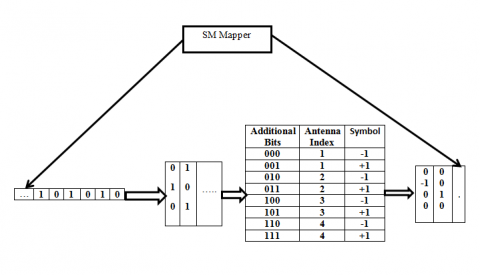Figure 2. Spatial modulation MIMO system using antenna index

3. Statistics of Nakagami Fading Channel

Nakagami-m fading channel is defined by Nakagami distribution where m defines the fading parameter  or the shape parameter . Nakagami-m distribution is fitted for land to mobile communication, indoor to mobile multipath propagation and radio links pertaining to ionosphere. The probability density function (PDF) of Nakagami-m fading channel given as

$\mathrm{f}(\alpha, m, \xi)=\frac{2 m^m \alpha^{2 m-1}}{\xi^m \Gamma m} e^{\left(-\frac{m \alpha^2}{\xi}\right)}$        (1)

where, $\xi=E\left[|\alpha|^2\right]$ is the variance, m is the shape parameter , $\Gamma$ is the gamma distributed instantaneous signal to noise (SNR) which is expressed as,

$P_\gamma^\gamma=\frac{m^m \gamma^{2 m-1}}{\bar{\gamma}^m \Gamma m} e^{\left(-\frac{m \gamma}{\bar{\gamma}}\right)} ; \gamma \geq 0$      (2)

where, the average SNR is given as

$\bar{\gamma}=\frac{\xi E_s}{N_0}$        (3)

4. Analysis Using Metric of Pairwise Error Probability

To analyze the metric of pairwise error probability for modulation scheme of binary phase shift keying, modulation orders of 2,4 and 8 are considered for spatial modulation MIMO systems. MIMO systems are used to improve the diversity, spectral efficiency and reliability as it will combat multipath fading. In multipath fading the spatial MIMO channel can be correlated  using space time orthogonal block code (STBC) with Rayleigh fading or Rician fading and uncorrelated as considered in this research paper. But the fact is that it leads to increased radio frequency (RF) chains which is not desirable due to space constraints. To reduce the radio frequency chain, spatial modulation is beneficial where bulkiness is reduced by using spatial modulation multiple input multiple output system. In spatial modulation MIMO system, there is requirement of single radio frequency chain and antenna index is used for selection of antenna by which the digital data signal can be transmitted using the selected antenna. At the receiver the combined digital data sequence is detected at the receiver . The antenna index is represented as

$a=\log _2 N_t$       (4)

where, $a$ is antenna index and $N_t$ represents the number of transmitter antennas. Further, based on antenna index digital modulated bits can be represented as

$v_b=\log _2 M$        (5)

where, $v_b$ is the modulated digital data. The received signal vector is given as,

$y=H x+w$         (6)

where, $y$, H, x and w denote the received signal vector, MIMO channel matrix, transmission data vector and additive white Gaussian noise (AWGN) vector. In the receiver, detection process is done which is mainly suboptimal detection and optimal detection. Under suboptimal detection the modulated bits and the antenna index are independently carried out, whereas in optimal detection simultaneous operation is done on modulated bits and antenna index.

4.1 Suboptimal detection

Suboptimal detection is a separate detection process in terms of index of the antenna and digitally modulated bit sequence. Suboptimal detection of antenna index and digitally modulated bit sequence are stated as 

$\hat{a}=\arg _a \max \left|h_a^H y\right|$        (7)

$v_{\hat{b}}=\arg _b \min \left\|h_{\hat{a}} v_b\right\|^2-2 \operatorname{Re}\left\{h_a^H y v_b^*\right\}$         (8)

4.2 Optimal detection

Optimal detection considers modulated bits and antenna index to be detected simultaneously. The optimal detection can be written as ,

$\left\{\hat{a}, v_{\hat{b}}\right\}=\arg _{a, b} \min \left\|h_a v_b\right\|^2-2 \operatorname{Re}\left\{h_a^H y v_b^*\right\}$         (9)

Proceeding further, considering $v_{S M}\left(a, v_b\right)$ as the transmitted bit sequence, $v_{S M}\left(\hat{a}, v_{\hat{b}}\right)$ as the detected bit sequence at the destination, whereas $v_b$ and $v_{\hat{b}}$ denote the digitally modulated bit sequence and estimated digitally modulated bit sequence. In spatially modulation MIMO system, a is the antenna index and $\hat{a}$ is the estimated antenna index. PEP is $P\left(v_{S M}\left(a, v_b\right) \rightarrow v_{S M}\left(\hat{a}, v_{\hat{b}}\right)\right)$ .

$P\left(v_{S M}\left(a, v_b\right) \rightarrow v_{S M}\left(\hat{a}, v_{\hat{b}}\right)\right)$$=P\left(\left\|h_{\hat{a}} v_{\hat{b}}\right\|^2-2 R_e\left\{h_a^H y v_b^*\right\}\right)$        (10)

$P\left(v_{S M}\left(a, v_b\right) \rightarrow v_{S M}\left(\hat{a}, v_{\hat{b}}\right)\right)$ \begin{aligned} & =P\left(\left\|y-h_{\widehat{a}} v_b\right\|^2\right. \left.\leq\left\|y-h_a v_b\right\|^2\right)\end{aligned}      (11)

$P\left(v_{S M}\left(a, v_b\right) \rightarrow v_{S M}\left(\hat{a}, v_{\hat{b}}\right)\right)$ \begin{aligned} & \leq P\left(\left\|h_a v_b-h_a v_{\hat{b}}\right\|_F-\|w\|_F\right. \left.\leq\|w\|_F\right)\end{aligned}    (12)

$P\left(v_{S M}\left(a, v_b\right) \rightarrow v S M\left(\hat{a}, v_{\hat{b}}\right)\right) \leq P\left(\left\|\left(\frac{h_a v_b-h_a v_{\hat{b}}}{2}\right)\right\|_F \leq\|w\| F\right)$         (13)

$P\left(v_{S M}\left(a, v_b\right) \rightarrow v_{S M}\left(\hat{a}, v_{\hat{b}}\right)\right) \leq Q\left(\sqrt{\frac{\gamma \|\left(h_a v_b-h_a v_b \|_F^2\right.}{2}}\right)$        (14)

where, γ is energy per spatially modulated bit sequence and the Q function is mathematically given as

$Q(x)=\frac{1}{\sqrt{2 \pi}} \int_x^{\infty} e^{-\frac{z^2}{x}} d z$        （15）

The overall pairwise error probability (PEP) of spatial modulation multiple input multiple output system using union bound can be formulated as,

$P_e \leq \sum P\left(v_{S M}\left(j, v_l\right) \rightarrow v_{S M}\left(\hat{\jmath}, v_{\hat{b}}\right)\right)$       （16）

$\mathrm{P}_{\mathrm{e}}=\sum_{\mathrm{a}, b} P\left(u_{S M}\left(a, v_b\right) \rightarrow u_{S M}\left(\hat{a}, v_{\hat{b}}\right)\right)$       （17）

5. Simulation Results

Simulation results are obtained for spatial modulation wireless systems in matrix laboratory (MATLAB) platform to assess the metric of pairwise error probability against signal to noise ratio (SNR). Nakagami-m fading channel is considered with shape parameter m for various modulation order M, transmitter antennas Nt and receiver antennas Nr. The following Table 1 gives the simulation parameters, metrics and its corresponding values for spatial modulation multiple input multiple output system.

Figure 3 shows the pairwise error probability against signal to noise ratio (SNR) with modulation order M=2,4 and 8 with shape factor m=0. The number of transmitter and receiver antennas are 2 and 2 respectively. To achieve a PEP of 10-1, modulation order of 2 takes 3.2dB, modulation order 4 takes 5.6dB and modulation order 8 takes 9dB. When the modulation order of the system increases it requires more signal to noise ratio for 2 transmitting antennas and 2 receiving antennas. Also, the diversity gain is 4 which is the product of the number of transmitting and receiving antennas which can be minimal improvement in terms of performance.

Table 1. Simulation parameters and its metrics

 Simulation parameter Metrics Values Data signal Binary Phase Shift Keying modulated signal Binary 0’s and 1’s Modulation Order M=2.4 and 8 2-PSK, 4-PSK and 8-PSK Fading channel Nakagami-m Fading Channel Complex Random Variables ShapeParameter (or)Fading Parameter m m=0.1 MIMO RF Chain Transmitter Antennas= Nt Receiver Antennas=Nr Nt=2.4; Nr=2.4; Evaluation Metric Pairwise Error Probability (PEP) 10-1; 10-2; 10-3;

## 42_3333.png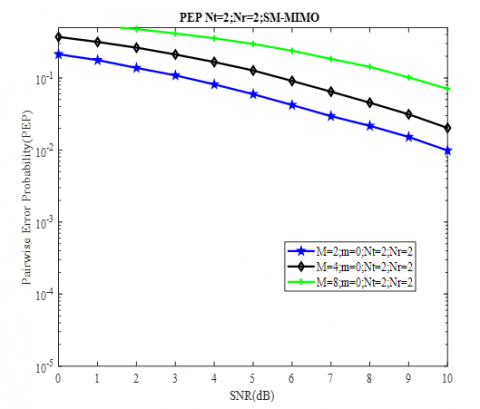Figure 3. Pairwise error probability versus SNR (dB) for shape factor m=0 and Nt =2and Nr=2 antennas

## 42_4444.png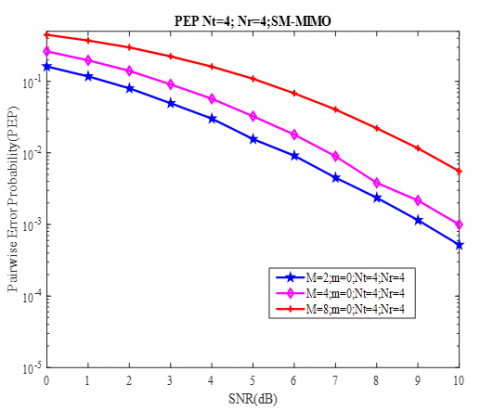Figure 4. Pairwise error probability versus SNR (dB) for shape factor m=0 and Nt =4and Nr=4 antennas

Figure 4 shows the pairwise error probability against signal to noise ratio with modulation order M=2,4 and 8 with shape factor m=0. The number of transmitter and receiver antennas are 4 and 4 respectively. To achieve a PEP of 10-2, modulation order of 2 takes 5.8dB, modulation order 4 takes 6.8dB and modulation order 8 takes 9.1dB. As per obtained results when the modulation order of the system increases the performance of the spatial modulation MIMO system gets reduced due to the fact a symbol has increased number of bits.

## 42_5555.png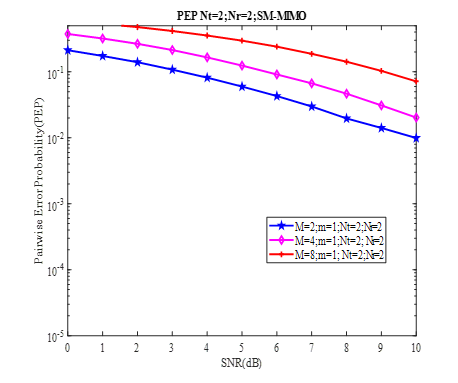Figure 5. Pairwise error probability versus SNR (dB) for shape factor m=1 and Nt =2and Nr=2 antennas

Figure 5 shows the performance when the shape factor is m=1 Nakagami-m fading channel for various modulation orders with 2 transmitter and 2 receiver antennas. To achieve a PEP of 10-1, modulation order of 2 takes 3.1dB, modulation order 4 takes 5.5dB and modulation order 8 takes 8.9dB. It infers when the shape parameter increases to 1 for Nakagami-m fading channel the SNR reduces by a significant value of 0.1dB in comparison to the results obtained in Figure 3.

## 42_6666.png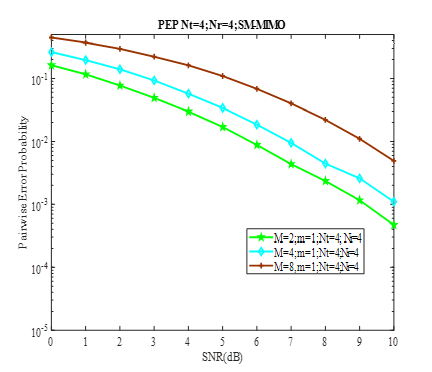Figure 6. Pairwise error probability versus SNR (dB) for shape factor m=1 and Nt =4and Nr=4 antennas

Figure 6 shows the performance when the shape factor is m=1 Nakagami-m fading channel for various modulation orders with 4 transmitter and 4 receiver antennas. To achieve a PEP of 10-2, modulation order of 2 takes 5.8dB, modulation order 4 takes 6.9 dB and modulation order 8 takes 9.1dB. It infers when the shape parameter increases to 1 for Nakagami-m fading channel the SNR reduces which implies that lesser power is needed for data signal transmission and reception. Further, medical signal such as electrocardiogram signal can be transmitted and received using spatial modulation MIMO systems.

6. Conclusions

Spatial modulation MIMO systems have profound interest towards reduction of RF chains. As in MIMO systems, there is requirement of large number of RF chain, bulkiness and complexity which gets reduced in spatial modulated MIMO system as compared to MIMO system. By virtue of the simulation results obtained in this research paper there is improvement in PEP for the lower order modulation M and significantly gets degraded for higher order modulation schemes in Nakagami-m fading channels in BPSK modulation scheme and its variant orders. Different antenna configurations have also been performed with respect to Nt transmitting and Nr receiving antennas. Further, development of upcoming 5G and 6G systems employing massive MIMO technologies and millimeter wave technologies can also employ spatial modulation which provide further research explorations.

References

 Wang, S., Li, W., Lei, J. (2018). Physical-layer encryption in massive MIMO systems with spatial modulation. China Communications, 15(10): 159-171. https://doi.org/10.1109/CC.2018.8485478

 Liu, P., Di Renzo, M., Springer, A. (2016). Line-of-sight spatial modulation for indoor mmwave communication at 60 GHz. IEEE Transactions on Wireless Communications, 15(11): 7373-7389. https://doi.org/10.1109/TWC.2016.2601616

 Sachan, V., Mishra, R.K. (2019). Uplink sum rate and capacity of hybrid precoding mmWave massive MIMO system. Traitement du Signal, 36(2): 155-160. https://doi.org/10.18280/ts.360205

 Alamouti, S. (1998). A simple transmit diversity technique for wireless communications. IEEE Journal on Selected Areas in Communications, 16(8): 1451-1458. https://doi.org/10.1109/49.730453

 Başar, E. (2016). Spatial modulation techniques for 5G wireless networks. 2016 24th Signal Processing and Communication Application Conference (SIU), Zonguldak, Turkey, pp. 777-780. https://doi.org/10.1109/SIU.2016.7495855

 Uluocak, S., Başar, E. (2017). Spatial modulation for multi-user massive MIMO systems. 2017 25th Signal Processing and Communications Applications Conference (SIU), Antalya, Turkey, pp. 1-4. https://doi.org/10.1109/SIU.2017.7960432

 Di Renzo, M., Haas, H., Ghrayeb, A., Sugiura, S., Hanzo, L. (2014). Spatial modulation for generalized MIMO: Challenges, opportunities, and implementation. Proceedings of the IEEE, 102(1): 56-103. https://doi.org/10.1109/JPROC.2013.2287851

 Fu, Y., Wang, C.X., Fang, X., Yan, L., Mclaughlin, S. (2020). BER performance of spatial modulation systems under a non-stationary massive MIMO channel model. IEEE Access, 8: 44547-44558. https://doi.org/10.1109/ACCESS.2020.2977139

 Younis, A., Serafimovski, N., Mesleh, R., Haas, H. (2010). Generalised spatial modulation. 2010 Conference Record of the Forty Fourth Asilomar Conference on Signals, Systems and Computers, Pacific Grove, CA, USA, pp. 1498-1502. https://doi.org/10.1109/ACSSC.2010.5757786

 Renzo, M.D., Haas, H., Grant, P.M. (2011). Spatial modulation for multiple antenna wireless systems: A survey. IEEE Communications Magazine, 49(12): 182-191. https://doi.org/10.1109/MCOM.2011.6094024

 Pillay, N., Xu, H. (2013). Comments on antenna selection in spatial modulation systems. IEEE Communications Letters, 17(9): 1681-1683. https://doi.org/10.1109/LCOMM.2013.072913.130801

 Jeganathan, J., Ghrayeb, A., Szczecinski, L. (2008). Spatial modulation: Optimal detection and performance analysis. IEEE Communications Letters, 12(8): 545-547. https://doi.org/10.1109/LCOMM.2008.080739

 Tarokh, V., Jafarkhani, H., Calderbank, A. (1999). Space-time block codes from orthogonal designs. IEEE Transactions on Information Theory, 45(5): 1456-1467. https://doi.org/10.1109/18.771146

 Gursoy, M.C., Poor, H.V., Verdu, S. (2005). The Noncoherent rician fading channel-Part-I: Structure of the capacity achieving input. IEEE Transactions on Wireless Communications, 4(5): 2193-2206. https://doi.org/10.1109/TWC.2005.853970

 Yip, K.W., Ng, T.S. (2000). A simulation model for Nakagami-m fading channels, m<1. IEEE Transactions on. Communications, 48(2): 214-221. https://doi.org/10.1109/26.823554

 Sachan, V., Kumar, I., Bhardwaj, L., Mishra, R.K. (2020). Pairwise error probability analysis of SM-MIMO system employing k-μ fading channel. Procedia Computer Science, 167: 2516-2523. https://doi.org/10.1016/j.procs.2020.03.304

 Zhou, Z., Ge, N., Lin, X. (2014). Reduced-complexity antenna selection schemes in spatial modulation. IEEE Communications Letters, 18(1): 14-17. https://doi.org/10.1109/LCOMM.2013.111413.131566

 Nguyen, T.D., Tran, X.N., Ngo, V.D., Le, M.T. (2015). A spatial modulation scheme with full diversity for four transmit antennas. 2015 International Conference on Advanced Technologies for Communications (ATC), Ho Chi Minh City, Vietnam, pp. 16-19. https://doi.org/10.1109/ATC.2015.7388310

 Tembhurne, A.P., Wyawahare, N.P. (2014). Alamouti based on spatial modulation for data transmission. International Conference for Convergence for Technology-2014, pp. 1-4. https://doi.org/10.1109/I2CT.2014.7092179

 Yang, P., Xiao, Y., Yu, Y., Li, S. (2011). Adaptive spatial modulation for wireless MIMO transmission systems. IEEE Communications Letters, 15(6): 602-604. https://doi.org/10.1109/LCOMM.2011.040711.110014

 Fan, S., Sun, Z., Xiao, Y., Zhou, X. (2019). Transmit antenna selection for spatial modulation OFDM Systems. 2019 IEEE 19th International Conference on Communication Technology (ICCT), Xi'an, China, pp. 800-804. https://doi.org/10.1109/ICCT46805.2019.8947030

 Yarkın, F., Altunbaş, İ. (2016). Outage probability of spatial modulation with antenna selection in Nakagami-m channel. 2016 24th Signal Processing and Communication Application Conference (SIU), Zonguldak, Turkey, pp. 885-888. https://doi.org/10.1109/SIU.2016.7495882

 Saleem, A.B., Hassan, S.A. (2020). On the performance of spatial modulation schemes in large-scale MIMO under correlated nakagami fading. 2020 IEEE 91st Vehicular Technology Conference (VTC2020-Spring), Antwerp, Belgium, pp. 1-5. https://doi.org/10.1109/VTC2020-Spring48590.2020.9128726

 Cogen, F., Aydin, E. (2020). Performance analysis of HQAM based spatial modulation over Nakagami-m fading channels. 2020 28th Signal Processing and Communications Applications Conference (SIU), Gaziantep, Turkey, pp. 1-4. https://doi.org/10.1109/SIU49456.2020.9302497

 Chen, Y.M., Lin, K.C., Peng, Y.H., Balaji., A., Li, C.P. (2021). A low-complexity high-rate spatial multiplexing aided generalized spatial modulation scheme. 2021 IEEE 32nd Annual International Symposium on Personal, Indoor and Mobile Radio Communications (PIMRC), Helsinki, Finland, pp. 434-439. https://doi.org/10.1109/PIMRC50174.2021.9569494

 Canbilen, A.E. (2022). Performance analysis of RIS-assisted spatial modulation over beckmann fading channels. 2022 30th Signal Processing and Communications Applications Conference (SIU), pp. 1-4. https://doi.org/10.1109/SIU55565.2022.9864941

 Wang, J., Jia, S., Song, J. (2012). Signal vector based detection scheme for spatial modulation. IEEE Communications Letters, 16(1): 19-21. https://doi.org/10.1109/LCOMM.2011.111611.112127

 Soria, F.R.C., Garcia, J.S., Abdala, V.I.R., Michel, R.P. (2015). Multiuser MIMO downlink transmission using spatial modulation. IEEE Latin America Transactions, 13(11): 3568-3572. https://doi.org/10.1109/TLA.2015 .7387932

 Simon, M.K., Alouini, M.S. (2000). Digital Communication over Fading Channels: A Unified Approach to Performance Analysis. John Wiley and Sons.

 Di Renzo, M., Haas, H. (2012). Bit error probability of SM- MIMO over generalized fading channels. IEEE Transactions on Vehicular Technology, 61(3): 1124-1144. https://doi.org/10.1109/TVT.2012.2186158

 Patel, S.B., Bhalani J., Trivedi, Y.N., (2020). Performance of full rate non-orthogonal STBC in spatially correlated MIMO systems. Radioelectronics and Communications Systems, 63(2): 88-95. https://doi.org/10.3103/S0735272720020041